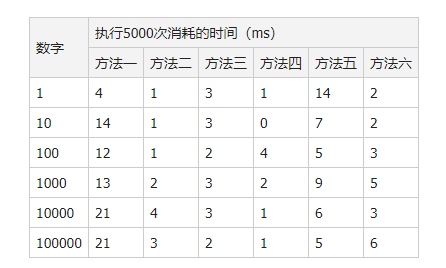# 关于JS实现的4种数字千位符格式化，例如“10,000”

26 篇文章 0 订阅

## 关于JS实现的4种数字千位符格式化

#### 属于转载，原网址，方便使用，这里记录一下

// 方法一
function toThousands(num) {
var result = [ ], counter = 0;
num = (num || 0).toString().split('');
for (var i = num.length - 1; i >= 0; i--) {
counter++;
result.unshift(num[i]);
if (!(counter % 3) && i != 0) { result.unshift(','); }
}
return result.join('');
}

// 方法二
function toThousands(num) {
var result = '', counter = 0;
num = (num || 0).toString();
for (var i = num.length - 1; i >= 0; i--) {
counter++;
result = num.charAt(i) + result;
if (!(counter % 3) && i != 0) { result = ',' + result; }
}
return result;
}

// 方法三
function toThousands(num) {
var num = (num || 0).toString(), re = /\d{3}$/, result = ''; while ( re.test(num) ) { result = RegExp.lastMatch + result; if (num !== RegExp.lastMatch) { result = ',' + result; num = RegExp.leftContext; } else { num = ''; break; } } if (num) { result = num + result; } return result; } 方法三是完全不同的算法，通过正则表达式循环匹配末尾的三个数字，每匹配一次，就把逗号和匹配到的内容插入到结果字符串的开头，然后把匹配目标（num）赋值为还没匹配的内容（RegExp.leftContext）。此外，还要注意： 1.如果数字的位数是3的倍数时，最后一次匹配到的内容肯定是三个数字，但是最前面的三个数字前不需要加逗号； 2.如果数字的位数不是3的倍数，那num变量最后肯定会剩下1到2个数字，循环过后，要把剩余的数字插入到结果字符串的开头。 虽然方法三减少了循环次数（一次循环处理三个字符），但由于用到了正则表达式，一定程度上增加了消耗。 方法四——方法三的字符串版 // 方法四 function toThousands(num) { var num = (num || 0).toString(), result = ''; while (num.length > 3) { result = ',' + num.slice(-3) + result; num = num.slice(0, num.length - 3); } if (num) { result = num + result; } return result; } 事实上，截取末尾三个字符的功能可以通过字符串类型的slice、substr或substring方法做到。这样就可以避免使用正则表达式。 方法五——分组合并法 // 方法五 function toThousands(num) { var num = (num || 0).toString(), temp = num.length % 3; switch (temp) { case 1: num = '00' + num; break; case 2: num = '0' + num; break; } return num.match(/\d{3}/g).join(',').replace(/^0+/, ''); } 先把数字的位数补足为3的倍数，通过正则表达式，将其切割成每三个数字一个分组，再通过join方法添加逗号，最后还要把补的0移除。 方法六——懒人法 // 方法六 function toThousands(num) { return (num || 0).toString().replace(/(\d)(?=(?:\d{3})+$)/g, '\$1,');
}1.对比方法一、二，每次操作3个字符而不是1个字符，减少循环次数；
2.对比方法三、五、六，没有使用正则表达式，减少了消耗。

08-13
11-1824908-10
11-11
02-272万+
11-021146
12-11419
12-11787
01-191270
01-12671
07-08199
07-08598
08-13195
06-16340
08-29347
06-28303
10-143184点击重新获取扫码支付余额充值